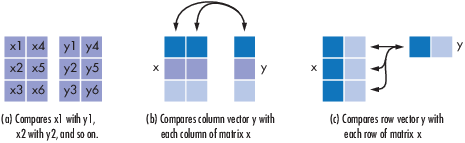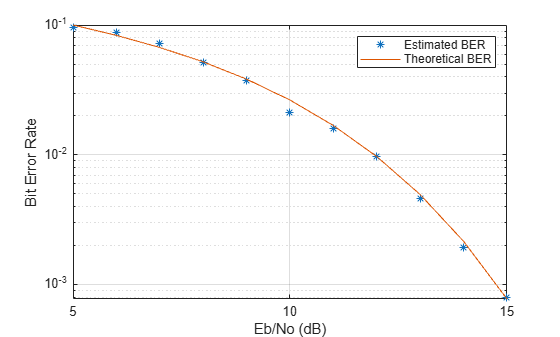Documentation

# biterr

Compute number of bit errors and bit error rate (BER)

## Syntax

```[number,ratio] = biterr(x,y) [number,ratio] = biterr(x,y,k) [number,ratio] = biterr(x,y,k,flg) [number,ratio,individual] = biterr(...) ```

## Description

### For All Syntaxes

The `biterr` function compares unsigned binary representations of elements in `x` with those in `y`. The schematics below illustrate how the shapes of `x` and `y` determine which elements `biterr` compares.Each element of `x` and `y` must be a nonnegative decimal integer; `biterr` converts each element into its natural unsigned binary representation. `number` is a scalar or vector that indicates the number of bits that differ. `ratio` is `number` divided by the total number of bits. The total number of bits, the size of `number`, and the elements that `biterr` compares are determined by the dimensions of `x` and `y` and by the optional parameters.

### For Specific Syntaxes

`[number,ratio] = biterr(x,y) ` compares the elements in `x` and `y`. If the largest among all elements of `x` and `y` has exactly k bits in its simplest binary representation, the total number of bits is k times the number of entries in the smaller input. The sizes of `x` and `y` determine which elements are compared:

• If `x` and `y` are matrices of the same dimensions, then `biterr` compares `x` and `y` element by element. `number` is a scalar. See schematic (a) in the preceding figure.

• If one is a row (respectively, column) vector and the other is a two-dimensional matrix, then `biterr` compares the vector element by element with each row (resp., column) of the matrix. The length of the vector must equal the number of columns (resp., rows) in the matrix. `number` is a column (resp., row) vector whose mth entry indicates the number of bits that differ when comparing the vector with the mth row (resp., column) of the matrix. See schematics (b) and (c) in the figure.

`[number,ratio] = biterr(x,y,k) ` is the same as the first syntax, except that it considers each entry in `x` and `y` to have `k` bits. The total number of bits is `k` times the number of entries of the smaller of `x` and `y`. An error occurs if the binary representation of an element of `x` or `y` would require more than `k` digits.

`[number,ratio] = biterr(x,y,k,flg) ` is similar to the previous syntaxes, except that `flg` can override the defaults that govern which elements `biterr` compares and how `biterr` computes the outputs. The possible values of `flg` are `'row-wise'`, `'column-wise'`, and `'overall'`. The table below describes the differences that result from various combinations of inputs. As always, `ratio` is `number` divided by the total number of bits. If you do not provide `k` as an input argument, the function defines it internally as the number of bits in the simplest binary representation of the largest among all elements of `x` and `y`.

Comparing a Two-Dimensional Matrix x with Another Input y

Shape of yflgType of ComparisonnumberTotal Number of Bits
2-D matrix `'overall'` (default) Element by element Total number of bit errors `k` times number of entries of `y`
`'row-wise'`mth row of `x` vs. mth row of `y` Column vector whose entries count bit errors in each row `k` times number of entries of `y`
`'column-wise'`mth column of `x` vs. mth column of `y` Row vector whose entries count bit errors in each column `k` times number of entries of `y`
Row vector `'overall'``y` vs. each row of `x` Total number of bit errors `k` times number of entries of `x`
`'row-wise'`(default) `y` vs. each row of `x` Column vector whose entries count bit errors in each row of `x` `k` times size of `y`
Column vector `'overall'``y` vs. each column of `x` Total number of bit errors `k` times number of entries of `x`
`'column-wise'` (default) `y` vs. each column of `x` Row vector whose entries count bit errors in each column of `x` `k` times size of `y`

`[number,ratio,individual] = biterr(...)` returns a matrix `individual` whose dimensions are those of the larger of `x` and `y`. Each entry of `individual` corresponds to a comparison between a pair of elements of `x` and `y`, and specifies the number of bits by which the elements in the pair differ.

## Examples

collapse all

Create two binary matrices.

`x = [0 0; 0 0; 0 0; 0 0]`
```x = 4×2 0 0 0 0 0 0 0 0 ```
`y = [0 0; 0 0; 0 0; 1 1]`
```y = 4×2 0 0 0 0 0 0 1 1 ```

Determine the number of bit errors.

`numerrs = biterr(x,y)`
```numerrs = 2 ```

Determine the number of errors computed column-wise.

`numerrs = biterr(x,y,[],'column-wise')`
```numerrs = 1×2 1 1 ```

Compute the number of row-wise errors.

`numerrs = biterr(x,y,[],'row-wise')`
```numerrs = 4×1 0 0 0 2 ```

Compute the number of overall errors. This has the same behavior as the default.

`numerrs = biterr(x,y,[],'overall')`
```numerrs = 2 ```

Demodulate a noisy 64-QAM signal and estimate the bit error rate (BER) for a range of Eb/No values. Compare the BER estimate to theoretical values.

Set the simulation parameters.

```M = 64; % Modulation order k = log2(M); % Bits per symbol EbNoVec = (5:15)'; % Eb/No values (dB) numSymPerFrame = 100; % Number of QAM symbols per frame```

Initialize the results vector.

`berEst = zeros(size(EbNoVec));`

The main processing loop executes the following steps:

• Generate binary data and convert to 64-ary symbols

• QAM modulate the data symbols

• Pass the modulated signal through an AWGN channel

• Demodulate the received signal

• Convert the demodulated symbols into binary data

• Calculate the number of bit errors

The `while` loop continues to process data until either 200 errors are encountered or 1e7 bits are transmitted.

```for n = 1:length(EbNoVec) % Convert Eb/No to SNR snrdB = EbNoVec(n) + 10*log10(k); % Reset the error and bit counters numErrs = 0; numBits = 0; while numErrs < 200 && numBits < 1e7 % Generate binary data and convert to symbols dataIn = randi([0 1],numSymPerFrame,k); dataSym = bi2de(dataIn); % QAM modulate using 'Gray' symbol mapping txSig = qammod(dataSym,M); % Pass through AWGN channel rxSig = awgn(txSig,snrdB,'measured'); % Demodulate the noisy signal rxSym = qamdemod(rxSig,M); % Convert received symbols to bits dataOut = de2bi(rxSym,k); % Calculate the number of bit errors nErrors = biterr(dataIn,dataOut); % Increment the error and bit counters numErrs = numErrs + nErrors; numBits = numBits + numSymPerFrame*k; end % Estimate the BER berEst(n) = numErrs/numBits; end```

Determine the theoretical BER curve using `berawgn`.

`berTheory = berawgn(EbNoVec,'qam',M);`

Plot the estimated and theoretical BER data. The estimated BER data points are well aligned with the theoretical curve.

```semilogy(EbNoVec,berEst,'*') hold on semilogy(EbNoVec,berTheory) grid legend('Estimated BER','Theoretical BER') xlabel('Eb/No (dB)') ylabel('Bit Error Rate')```